# Introduction

This document demonstrates how to use the soilDB package to download data from the Henry Mount soil climate database. Soil climate data are routinely collected by SSO staff via buried sensor/data-logger devices (“hobos”) and now above ground weather stations. The Henry Mount Soil Climate database was established to assist with the management and analysis of these data.

# Setup R Environment

With a recent version of R (>= 2.15), it is possible to get all of the packages that this tutorial depends on via:

``````# run these commands in the R console
install.packages('RColorBrewer', dep=TRUE)
install.packages('latticeExtra', dep=TRUE)
install.packages('reshape', dep=TRUE)
install.packages('dismo', dep=TRUE)
install.packages('soilDB', dep=TRUE)
install.packages('sharpshootR', dep=TRUE)
install.packages('devtools', dep=TRUE)

# Getting and Viewing Data

Soil climate data can be queried by:

• project (typically a soil survey area, “CA630”)
• NASIS user site ID (e.g. “2006CA7920001”)
• MLRA soil survey office (e.g. “2-SON”)

and optionally filtered by:

• start date (“YYYY-MM-DD”)
• end date (“YYYY-MM-DD”)
• sensor type:
• “all”: all available time series data
• “soiltemp”: soil temperature time series
• “soilVWC”: soil volumetric water content time series
• “airtemp”: air temperature time series
• “waterlevel”: water level time series

and aggregated to the following granularity:

• “hour” (houly data are returned if available)
• “day” (MAST and mean summer/winter temperatures are automatically computed)
• “week”
• “month”
• “year”

Query daily sensor data associated with the Sequoia / Kings Canyon soil survey.

``````library(soilDB)
library(sharpshootR)
library(latticeExtra)
library(RColorBrewer)
library(plyr)

# get soil temperature, soil moisture, and air temperature data
x <- fetchHenry(project='CA792')

# check object structure:
str(x, 2)``````

Quick listing of essential site-level data. “Functional years” is the number of years of non-missing data, after grouping data by Day of Year. “Complete years” is the number of years that have 365 days of non-missing data. “dslv” is the number of days since the data-logger was last visited.

``````# convert into data.frame
d <- as.data.frame(x\$sensors)
# keep only information on soil temperature sensors at 50cm
d <- subset(d, subset=sensor_type == 'soiltemp' & sensor_depth == 50)
# check top 6 rows and select columns``````
user_site_id name sensor_depth MAST Winter Summer STR functional.yrs complete.yrs dslv
2006CA7920001 Muir Pass 50 1.40 -1.33 4.88 cryic 11 10 1800
2012CA7921062 Dusy Basin 50 4.84 1.04 10.20 cryic 4 2 1801
2015CA7921071 Tyndall 50 5.68 1.17 11.61 cryic 3 2 1763
S2012CA019001 Littlepete 50 4.93 1.30 9.75 cryic 6 5 1801
S2012CA019002 LeConte 50 6.28 1.66 11.69 cryic 6 5 1800
S2012CA019003 McDermand 50 3.91 0.93 8.09 cryic 6 5 1800
The tables with sensor data (e.g. `x\$soiltemp`) look like this:
sid date_time sensor_value year doy month season water_year water_day name sensor_name sensor_depth
26 2005-12-31 16:00:00 NA 2006 1 Dec Winter 2006 93 Muir Pass-50 Muir Pass 50
26 2006-01-01 16:00:00 NA 2006 2 Jan Winter 2006 94 Muir Pass-50 Muir Pass 50
26 2006-01-02 16:00:00 NA 2006 3 Jan Winter 2006 95 Muir Pass-50 Muir Pass 50
26 2006-01-03 16:00:00 NA 2006 4 Jan Winter 2006 96 Muir Pass-50 Muir Pass 50
26 2006-01-04 16:00:00 NA 2006 5 Jan Winter 2006 97 Muir Pass-50 Muir Pass 50
26 2006-01-05 16:00:00 NA 2006 6 Jan Winter 2006 98 Muir Pass-50 Muir Pass 50

Make a simple graphical timeline of the data by sensor.

``HenryTimeLine(x\$soiltemp, main='Soil Temperature Records', col='RoyalBlue')``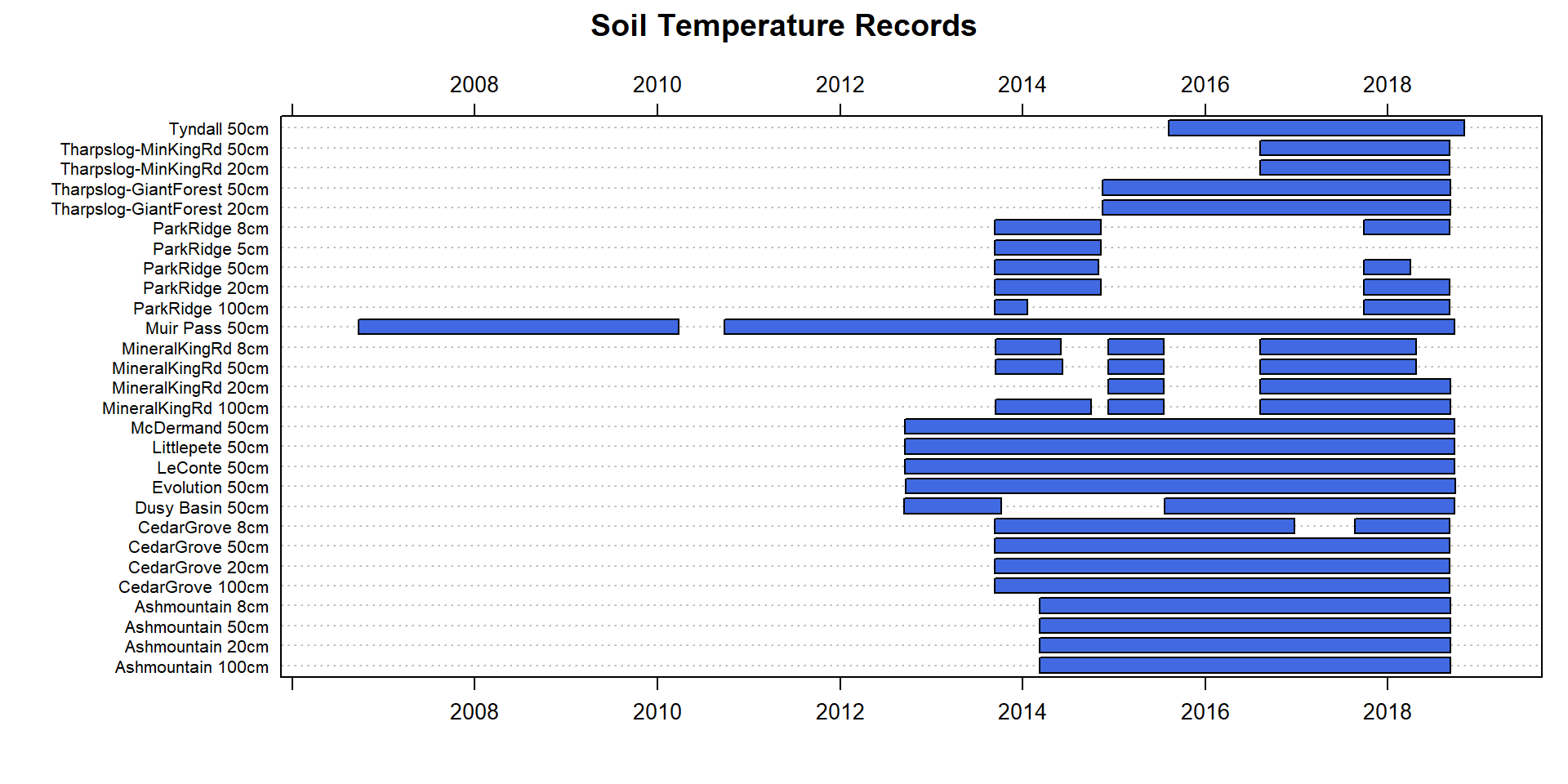``HenryTimeLine(x\$soilVWC, main='Soil VWC Records', col='RoyalBlue')``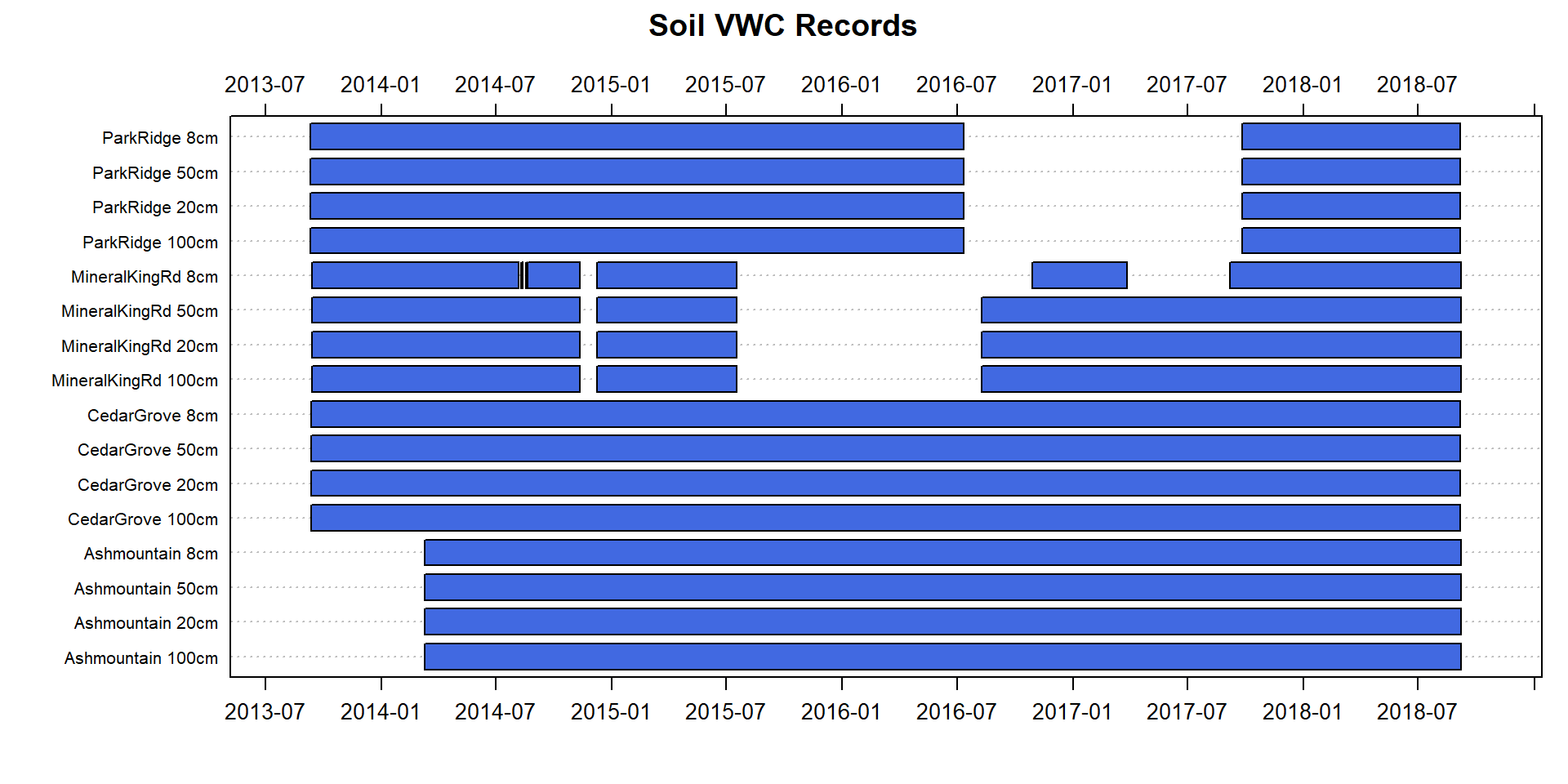## Plot Data

All of the following examples are based on lattice graphics. The syntax takes a little getting used to, but provides a very flexible framework for layout of grouped data into panels. Try adapting the examples below as a starting point for more complex or customized figures. Critical elements of the syntax include:

• formula interface: `sensor_value ~ date_time | sensor_name`: y ~ x | facet-by-groups
• data source: `data=x\$soiltemp`: get data from the soiltemperature sensor records
• optional subsetting: `subset=sensor_depth == 50`: keep only records at 50cm
``````# soil temperature at 50cm
xyplot(sensor_value ~ date_time | sensor_name,
data=x\$soiltemp, subset=sensor_depth == 50,
main='Daily Soil Temperature (Deg. C) at 50cm', type=c('l', 'g'),
as.table=TRUE, xlab='Date', ylab='Deg C',
scales=list(alternating=3, cex=0.75, x=list(rot=45)),
strip=strip.custom(bg='grey')
)``````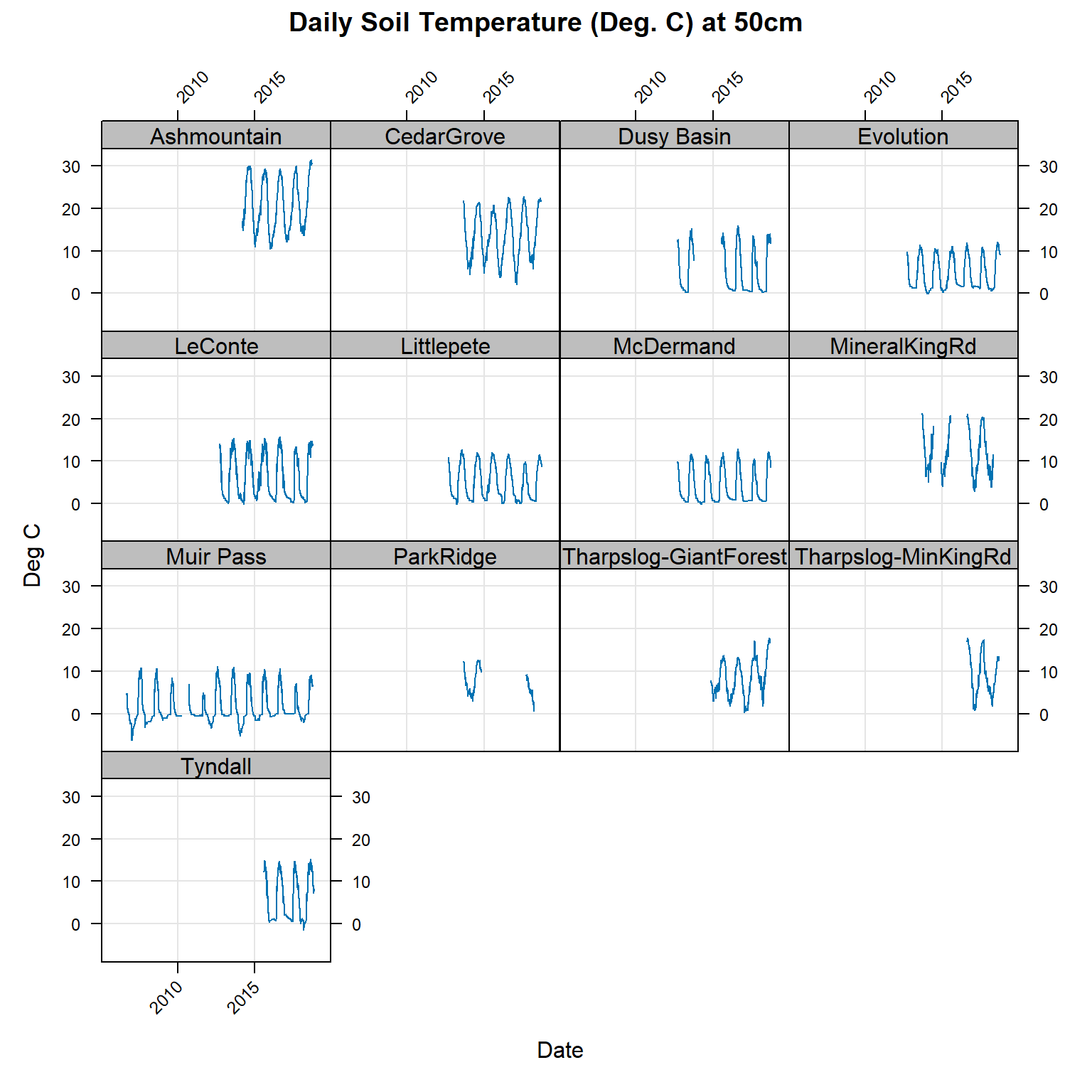``````xyplot(sensor_value ~ date_time | sensor_name,
data=x\$soilVWC, subset=sensor_depth == 50,
main='Daily Soil Moisture at 50cm', type=c('l', 'g'),
as.table=TRUE, xlab='Date', ylab='Volumetric Water Content',
scales=list(alternating=3, cex=0.75, x=list(rot=45)),
strip=strip.custom(bg='grey')
)``````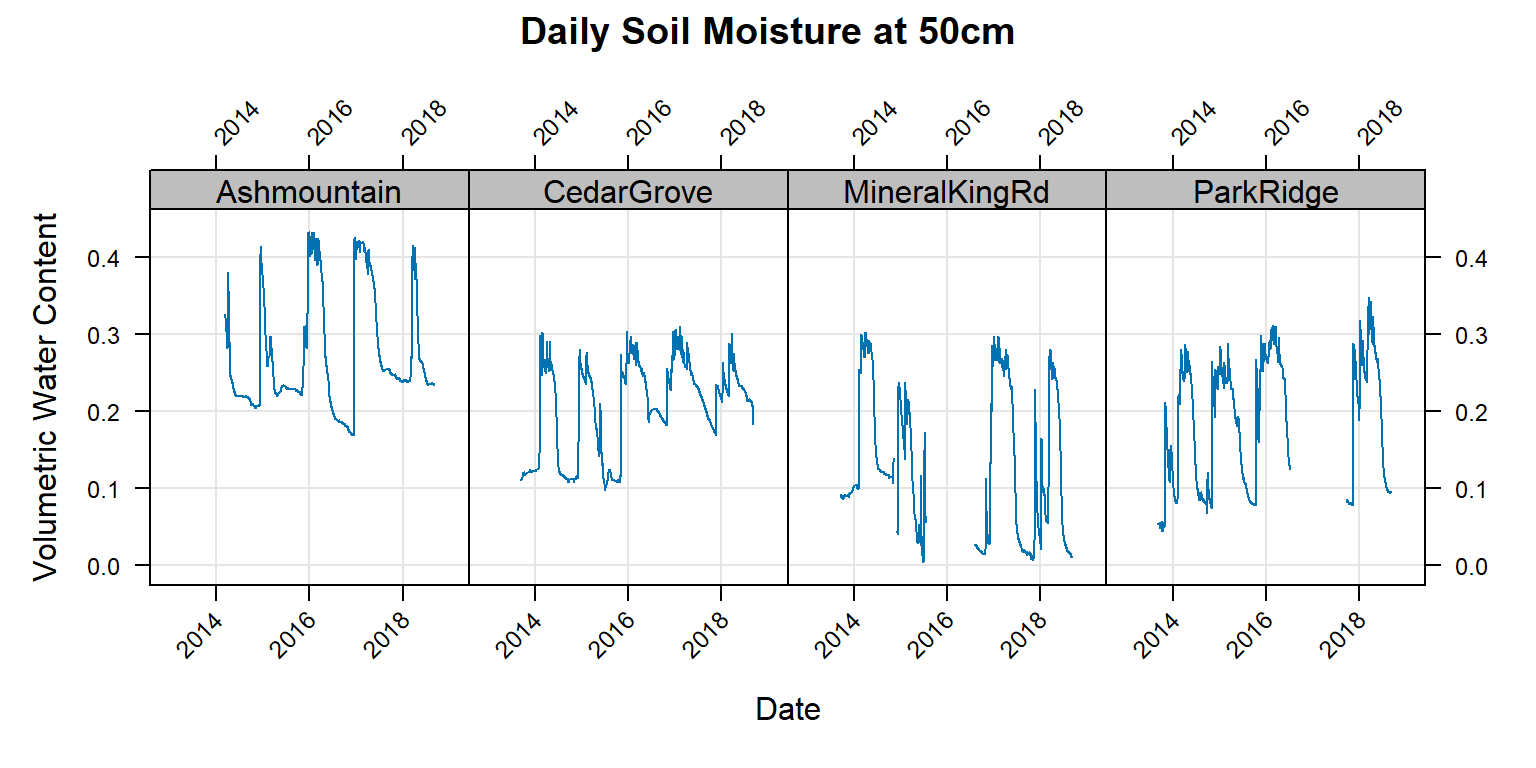One approach for investigating data gaps, blue: data, grey: no data.

``````levelplot(factor(!is.na(sensor_value)) ~ doy * factor(year) | sensor_name,
data=x\$soiltemp,
subset=sensor_depth == 50,
main='Daily Soil Temperature (Deg. C) at 50cm',
col.regions=c('grey', 'RoyalBlue'), cuts=1,
colorkey=FALSE, as.table=TRUE, scales=list(alternating=3, cex=0.75),
par.strip.text=list(cex=0.85), strip=strip.custom(bg='yellow'),
xlab='Day of Year', ylab='Year')``````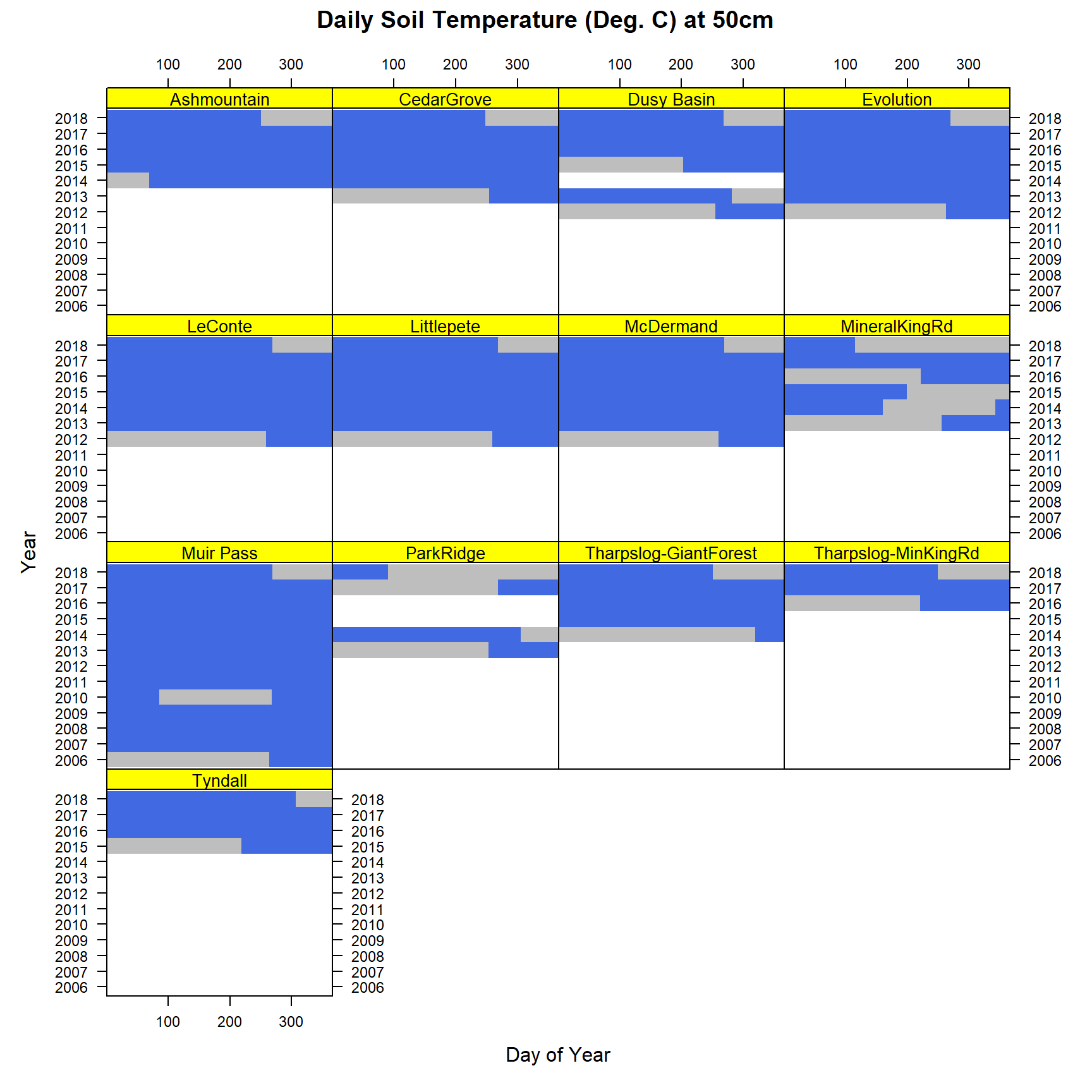Again, this time only include 2013-2017.

``````levelplot(factor(!is.na(sensor_value)) ~ doy * factor(year) | sensor_name,
data=x\$soiltemp,
subset=sensor_depth == 50 & year %in% 2013:2017,
main='Daily Soil Temperature (Deg. C) at 50cm',
col.regions=c('grey', 'RoyalBlue'), cuts=1,
colorkey=FALSE, as.table=TRUE, scales=list(alternating=3, cex=0.75),
par.strip.text=list(cex=0.85), strip=strip.custom(bg='yellow'),
xlab='Day of Year', ylab='Year')``````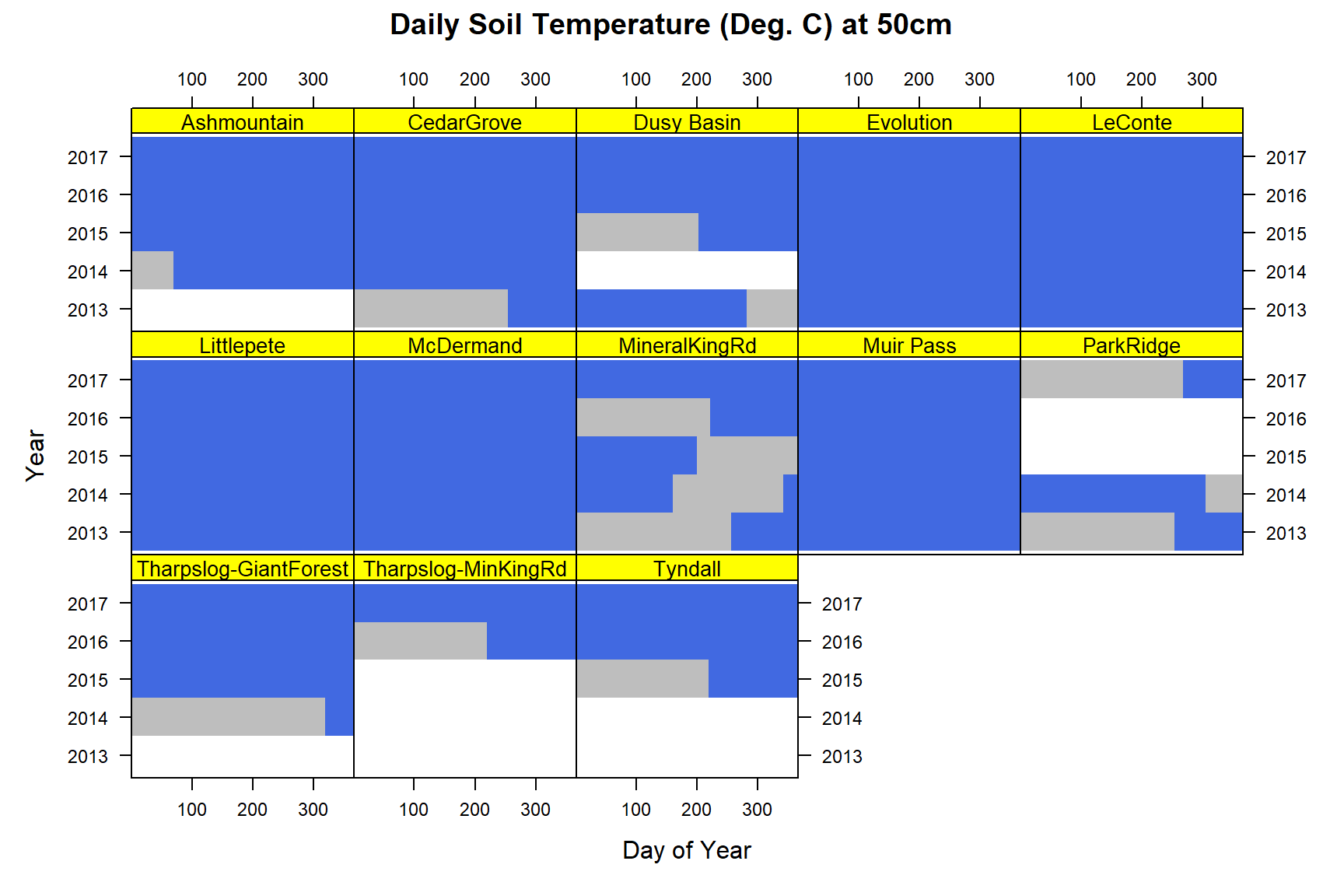Soil moisture data by calendar years and Julian day.

``````levelplot(factor(!is.na(sensor_value)) ~ doy * factor(year) | sensor_name, main='Daily Soil Moisture at 50cm',
data=x\$soilVWC, subset=sensor_depth == 50,
col.regions=c('grey', 'RoyalBlue'), cuts=1,
colorkey=FALSE, as.table=TRUE, scales=list(alternating=3, cex=0.75),
par.strip.text=list(cex=0.85), strip=strip.custom(bg='yellow'),
xlab='Day', ylab='Year')``````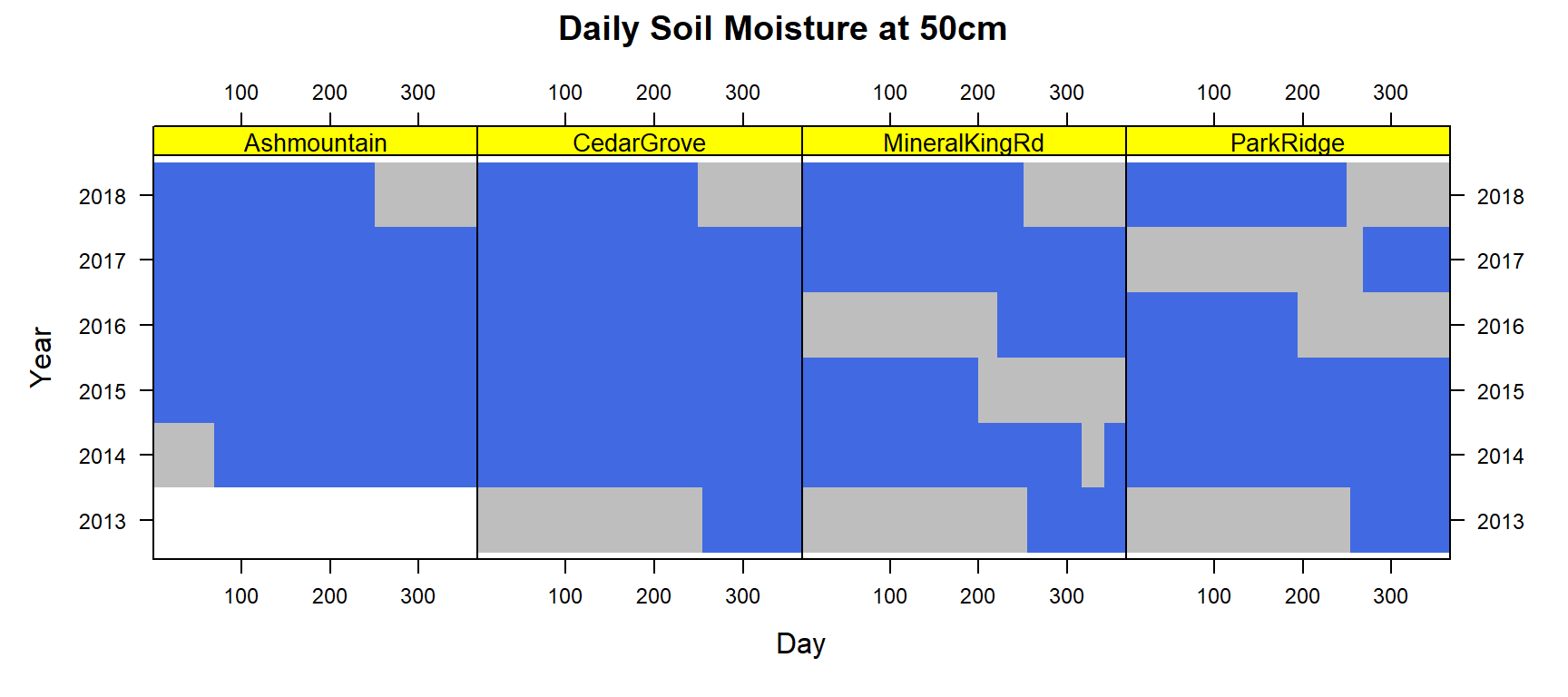Soil moisture data by water year and day.

``````levelplot(factor(!is.na(sensor_value)) ~ water_day * factor(water_year) | sensor_name, main='Daily Soil Moisture at 50cm\nOctober 1 -- September 30',
data=x\$soilVWC, subset=sensor_depth == 50,
col.regions=c('grey', 'RoyalBlue'), cuts=1,
colorkey=FALSE, as.table=TRUE, scales=list(alternating=3, cex=0.75),
par.strip.text=list(cex=0.85), strip=strip.custom(bg='yellow'),
xlab='Water Day', ylab='Water Year')``````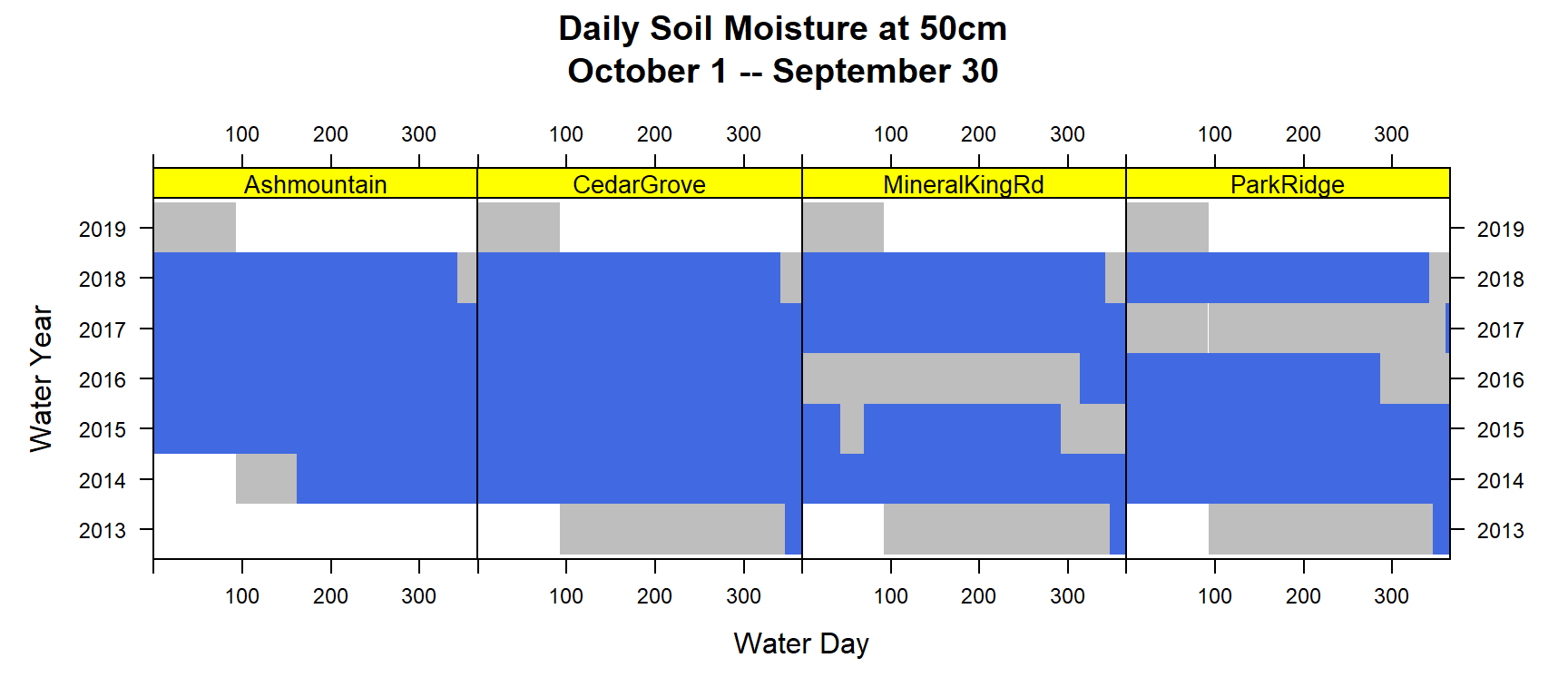Comparison between years, faceted by sensor name.

``````# generate some better colors
cols.temp <- colorRampPalette(rev(brewer.pal(11, 'RdYlBu')), space='Lab', interpolate='spline')

levelplot(sensor_value ~ doy * factor(year) | sensor_name, main='Daily Soil Temperature (Deg. C) at 50cm',
data=x\$soiltemp, col.regions=cols.temp,
subset=sensor_depth == 50 & year %in% 2013:2017,
colorkey=list(space='top'), as.table=TRUE, scales=list(alternating=3, cex=0.75),
par.strip.text=list(cex=0.85), strip=strip.custom(bg='grey'),
xlab='Day of Year', ylab='Year')``````